CHAPTER 3

CONSTRUCTION OF THE MAPS

SEVEN or more mechanisms, described in Chapter 2, contribute to the deformation of crystalline solids. The one which is dominant (meaning that it contributes most to the total rate of deformation) depends on the stress and temperature to which the solid is exposed, and on its properties. It is helpful, for a given material, to have a way of plotting the field of dominance of each mechanism and of displaying both the experimental data and the pre­dictions that the model-based equations of Section 2 make for it. Such a diagram (or "map") sum­marizes in a compact form both the experimental and model-based understanding of the materials (Ashby, 1972 ; Frost and Ashby, 1973 ).

Ways of doing this were introduced briefly in Chapter 1, and illustrated, for nickel, by Figs. 1.2 to 1.6. For the reasons given there, it is preferable to use as axes, the macroscopic variables σs, T/TM,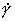, and, when discussing non-steady-state behaviour (Chapter 17, Section 17.1), the strain γ, or the time t. Of the possible combinations, we have found that with axes of σs and T/TM (Fig. 1.2) and that with axes ofand σs (Fig. 1.3) are the most useful: the first covers the full range of all the macroscopic variables, and best allows comparison of theory and experiment at low temperatures, while the second displays the creep regime, and permits accurate comparison of theory and experiment at high temperatures. They appear throughout Chapters 4 to 16. The map with axes ofand T/TM has merit for displaying behavior at very high strain rates (Chapter 17, Section 17.2).

A deformation map for a material is constructed by the following procedure. Examples of each step will be found throughout Chapters 4 to 16.

First, data for the material properties are gathered: lattice parameter, molecular volume, and Burger's vector; moduli and their temperature dependencies; and lattice, boundary and core diffusion coefficients (if they exist). It is often necessary to replot data for moduli and diffusion coefficients in order to make a sensible choice of constants, μ0, dμ/dT, D, Qυ, δD0b, Qb, etc.

Second, data for the hardness, low-temperature yield, and creep are gathered: flow strength as a function of temperature and strain rate, and creep rate as a function of temperature and stress. These data are plotted on transparent paper with the axes used for the maps themselves: log10 (σs) and T/TM, or log10 () and log10 (σs). Each datum is plotted as a symbol which identifies its source, and is labeled with the value of the third macroscopic variable: log10 () or T.

Third, an initial estimate is made of the material properties describing glide (F,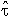, Fp,p, etc.) and creep (n, A, etc.) by fitting eqns. (2.9), (2.12) and (2.21) to these data plots. From the plots it is also possible to make an initial estimate of the stress at which the simple power-law for creep breaks down, giving α' of eqn. (2.26).

Fourth, the initial values for the material pro­perties are used to construct a trial map. This is best done by a simple computer program which steps incrementally through the range of σsand T/TM (orand σs), evaluating and summing the rate-equations at each step, and which plots the result in the forms shown in Figures which appear in Chapter 1.

All the maps (regardless of the choice of axes) are divided into fields, within each of which a given mechanism is dominant. The field boundaries  are the loci of points at which two mechanisms contribute equally to the overall strain-rate, and are computed by equating pairs (or groups) of rate-equations, and solving for stress as a function of temperature as shown in Fig. 3.1. Superimposed on this are the contours of constant strain-rate, obtained by summing the rate-equations in an appropriate way (discussed below) to give a total strain-rate,net and plotting the loci of points for whichnet has given constant values, as shown in Fig. 1.2.

Fifth, the data plots are laid over the trial maps, allowing the data to be divided into blocks accord­ing to the dominant flow mechanism. It is then possible to make a detailed comparison between each block of data and the appropriate rate equation. The material properties are now adjusted to give the best fit between theory and experimental data. New maps are now computed and the comparison repeated. Final adjustments are made by constructing maps of the types described in Chapter 1, plotting the data onto them, and examining both goodness-of-fit in individual fields and the precision with which computed and experimental field boun­daries coincided. It cannot be emphasized too strongly that for the final map to have any real value, this detailed comparison with data over the entire range T, σs andis essential.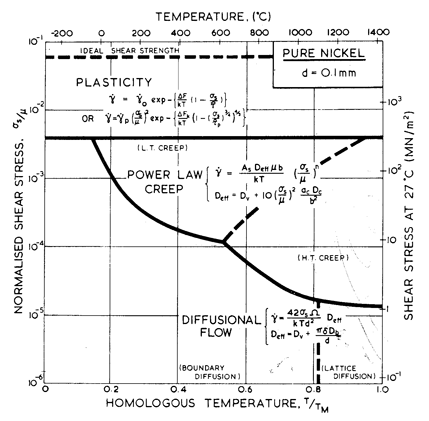Fig. 3.1. The construction of a deformation-mechanism map. The field boundaries are the loci of points at which two mechanisms (or combinations of mechanisms—see text) have equal rates.

Finally, the adjusted data are tabulated and the maps redrawn, either with data plotted on them, or on separate data-plots. Such tables and plots for each material discussed in this book will be found in Chapters 4 to 16.

 3.2 SUPERPOSITION OF RATE-EQUATIONS

The method of combining the rate-equations requires some discussion. Glide plasticity is de­scribed by two rate-equations (Section 2.2), one for obstacle-controlled glide (2, eqn. (2.9)) and one for lattice-resistance-controlled glide (3, eqn. (2.12)). At the lowest level of approximation, they can be treated as alternatives:plas = Least of {2,3} (3.1)

This is the level adopted here. It is equivalent to assuming that the strongest obstacles control the flow stress, and is entirely adequate for our purposes.

A better approximation is to recognize that, when several strengthening mechanisms (drag, discrete obstacles, lattice resistance) operate at once, their contributions to the flow stress superimpose in a roughly linear way. Even this is an approximation; the superposition is seldom truly linear. The highest precision is possible only by modeling the detailed way in which a given pair of mechanisms interact (see Evans and Rawlings, 1969 ; Kocks et al., 1975 ; Frost and Ashby, 1971 ).

Power-law creep (4, eqn. (2.21) or6, eqn. (2.26)) and diffusional flow (7, eqn. (2.29)) are independent flow mechanisms involving different defects. To a first approximation, their strain-rates add. Power­law creep (4) and glide (plas eqn. (3.1)) do not. Both processes involve the same defect; they de­scribe the same dislocations moving under different conditions. As the stress is raised, the gliding part of the motion of a dislocation becomes more impor­tant, and the climbing part less so until, when the boundary between the two fields is reached, power­law climb is not necessary at all. We have solved the problem by treating power-law creep and glide plasticity as alternative mechanisms, choosing always the faster one. This divides the map into two parts, one ("power-law creep") depicting steady-rate flow and one ("plasticity") depicting flow at cons­tant structure—a consequence of the fact that the glide equations do not include recovery, and there­fore cannot properly describe the transition from constant-structure to glide-controlled plasticity at steady state. Harper-Dorn Creep (5, eqn. (2.23)) is treated as an alternative to diffusional flow, again selecting the faster mechanism. Finally, if the ideal strength is exceeded, flow (l. eqn. (2.1)) becomes catastrophic. In summary, the net strain rate of a polycrystal subject to a stress as at a temperature T is:net =1 + greatest of (plas,4 or6)  + greatest of (5,7) (3.2)

Within a field, the contribution of one mechanism tonet is larger than any other. It is separated by field boundaries (heavy, full lines) from fields of dominance of other mechanisms. The power-law creep equation (2.21) and the diffusional flow equation (2.29) each describe the sum of two additive contributions; heavy broken lines separate the regions of dominance of each contribution. The contours of constant strain rate are obtained by solving eqn. (3.2) for  as a function of T at constantnet.

 3.3 TREATMENT OF DATA

The origins and detailed treatment of data are described under the headings of the individual materials. As emphasized earlier, the accuracy of the maps reflects that of the experiments. Different experimenters often report strain-rates that differ by a factor as large as 100 at a given σs and T; it is necessary to judge which experiments more accurately reflect the true material behavior. These judgments are to some extent subjective because of the large number of variables involved: purity, testing atmosphere, grain size, thermomechanical history, recrystallization effects, type of test, and so forth. The effect of impurities is most pronounced in nominally "pure" metals: as little as 0.1% impurity lowers the creep-rate of pure nickel by more than an order of magnitude (Dennison et al., 1966 ). The low-temperature yield stress of b.c.c. metals is raised substantially by even smaller amounts of interstitial impurities. Grain size (if not extreme) atmosphere, and test type are less important, but, when relevant, we have tried to record them (grain size appears explicitly on all maps). Dynamic recrystallization can be a problem; it can cause the strain-rate to oscillate (at constant stress) or the flow stress to oscillate (at constant strain-rate) and makes it difficult to define steady-state behavior. If recrystallization (which depends dramatically on purity) occurs only once during a test, it may be neglected in evaluating steady-rate behavior. But if the test produces repeated recrystallization, as is common in hot torsion tests taken to large strains, the suc­cessive waves of recrystallization may overlap to produce another type of steady-state behavior. This regime is shown as a shaded region on the maps.

As mentioned above, the parameters appearing in the rate-equations are adjusted to give the best description of the experimental data. Most of the adjustment involves the dislocation creep parameters, n and A and the dislocation glide parameters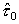,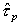and ∆Fp. In some cases the core diffusion co­efficients have been adjusted to fit low-temperature creep data.

The data on which each map is based are plotted on the map or as a separate data plot. Points attached by solid lines have the same strain-rate. A dashed line between points indicates a series of intermediate experimental points. Included on the plots are creep, tension, compression, torsion and (occasionally) extrusion tests, all converted to shear stress. Torsion and extrusion data present difficulties because the stress and strain-rate are not constant throughout the specimen; they must be inferred from a flow-field. The data for single crystals are different: they are plotted as critical resolved shear stress whenever possible, and with the standard conversion from tensile to shear stress (σs = σ/√3) otherwise. To compare them with the polycrystal data shown on the same map, the reader must multiply the single-crystal stresses by the appropriate factor: 1.77 for f.c.c. metals, for instance, and 1.67 for b.c.c. (see Chapter 2, Section 2.2 for further information on Taylor factors). In arriving at the optimized data of the tables shown in later chapters, single-crystal data were treated in this way.

Maps with normalized stress σs and temperature T/TM have, plotted on them, near the bottom, a line of constant shear stress (usually σs = 0.1 MN/m2). This allows the scale on the right-hand edge of the maps (of shear stress at 300 K) to be translated to give the shear stress at any temperature. All maps with T/TM as an axis carry, additionally, a scale of temperature in °C.

 References for Chapter 3

1.         Ashby, M.F., A first report on deformation-mechanism maps. Acta Metallurgica (pre 1990), 1972. 20: p. 887.

2.         Frost, H.J. and M.F. Ashby, A Second Report on Deformation-Mechanism Maps. 1973, Division of Applied Physics, Harvard University.

3.         Evans, A.G. and R.D. Rawlings, Thermally activated deformation of crystalline materials. Phys. Stat. Sol., 1969. 34: p. 9-31.

4.         Kocks, U.F., A.S. Argon, and M.F. Ashby, Prog. Mat. Sci., 1975. 19: p. 1.

5.         Frost, H.J. and M.F. Ashby, Motion of a dislocation acted on by viscous drag on an array of discrete obstacles. J. Appl. Phys, 1971. 42: p. 5273-9.

6.         Dennison, J.P., R.J. Llewellyn, and B. Wilshire, The Creep and Fracture Behaviour of Some Dilute Nickel Alloys at 500 and 6000 C. J. Inst. Met., 1966. 94: p. 130-34.